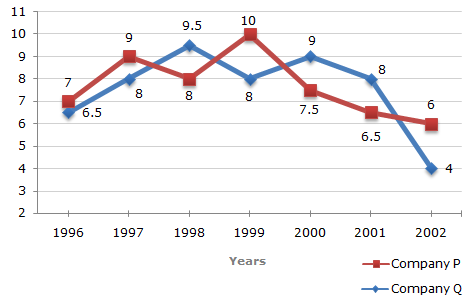# Data Interpretation - Line Charts - Discussion

### Discussion :: Line Charts - Line Chart 8 (Q.No.1)

Two different finance companies declare fixed annual rate of interest on the amounts invested with them by investors. The rate of interest offered by these companies may differ from year to year depending on the variation in the economy of the country and the banks rate of interest. The annual rate of interest offered by the two Companies P and Q over the years are shown by the line graph provided below.

Annual Rate of Interest Offered by Two Finance Companies Over the Years.1.

A sum of Rs. 4.75 lakhs was invested in Company Q in 1999 for one year. How much more interest would have been earned if the sum was invested in Company P?

 [A]. Rs. 19,000 [B]. Rs. 14,250 [C]. Rs. 11,750 [D]. Rs. 9500

Explanation:

 Difference = Rs. [(10% of 4.75) - (8% of 4.75)] lakhs = Rs. (2% of 4.75) lakhs = Rs. 0.095 lakhs = Rs. 9500.

 Sony said: (Apr 16, 2014) How 2%of 4.75 becomes 0.095?

 Praveen said: (Jul 29, 2014) 2% of 4.75 = 2/100 x 4.75 = 0.095.

 Zon said: (Jul 16, 2015) What is "Rs and lakhs"?

 Ugyen Zangmo said: (Aug 6, 2016) Why do we minus 8% from 10%?

 Kavi said: (Feb 6, 2017) @Sony. 2/100 is multiplied with 4.75. So 2*4.75 gives 9.5 then 9.5/100 is .095.

 Sweety said: (Dec 19, 2017) How first step came?

 Manas M said: (Aug 26, 2021) 10 * 4.75-8 * 4.75 = 9.5.

 Deki Bhutan said: (Aug 29, 2021) P= 10% of 4.75, Q= 8% of 4.75. 10% - 8% = 2% of 4.75, 2%/100 * 4.75 = 0.095. = 9500.